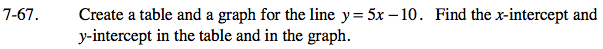### Home > AC > Chapter 7 > Lesson 7.2.2 > Problem7-67

7-67.

Create a table and a graph for the line y = 5x − 10. Find the x-intercept and y-intercept in the table and in the graph. 7-67 HW eTool (Desmos). Homework Help ✎Complete the table in the eTool below to determine the x and y-intercepts.
Click the link at right for the full version of the eTool: AC 7-67 HW eTool

Make a table substituting the x-values into the equation to find the y-values.

Plot the points.

The y-intercept is the value of y when x is 0, and the x-intercept is the value of x when y is 0.
Write the intercepts as ordered pairs.